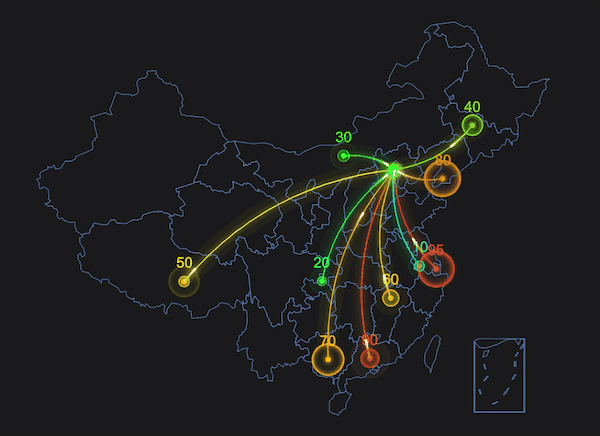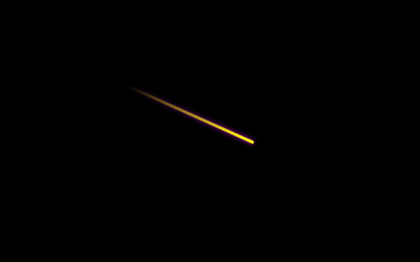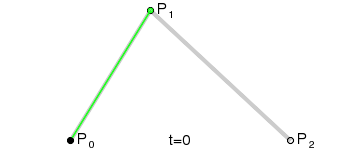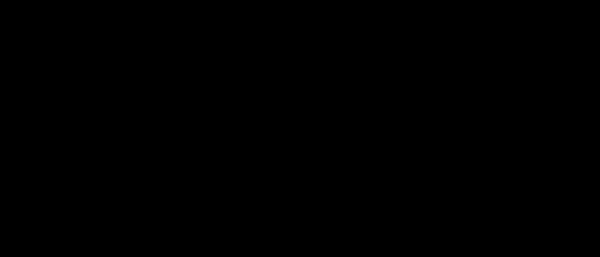# 使用 Canvas 绘制光线飞过效果``````<html lang="en">
<title>canvas 飞线</title>
<style>
body {
margin: 0;
background: #000000;
}

canvas {
position: absolute;
top: 0;
left: 0;
width: 100%;
height: 100%;
}
</style>

<body>
<canvas id="mycanvas"></canvas>
<script type="text/javascript">
const mycanvas = document.getElementById("mycanvas");
const context = mycanvas.getContext("2d");

const canvasWidth = window.innerWidth;
const canvasHeight = window.innerHeight;

mycanvas.width = canvasWidth;
mycanvas.height = canvasHeight;
</script>
</body>
</html>
``````

## 绘制光线尾迹``````// 创建渐变
function createGradient(context, p0, p1) {
const gradient = context.createLinearGradient(p0.x, p0.y, p1.x, p1.y);

}
``````

`p0``p1` 分别为线段的开始点和结束点，我们使用 `moveTo``lineTo` 绘制直线，并使用已创建好的渐变色来填充直线的路径，需要注意的是，这里的路径不需要闭合。

``````// 绘制线条
function createLine(p0, p1) {

context.beginPath();
context.moveTo(p0.x, p0.y);
context.lineTo(p1.x, p1.y);
context.lineCap = "round";
context.lineWidth = 3;
context.shadowColor = "rgba(255, 0, 255, 1)";
context.stroke();
}
``````

## 实现飞线动画

``````const P0 = {
x: 100,
y: 100,
};

const P1 = {
x: 600,
y: 300,
};
``````

``````x = P0.x + (P1.x - P0.x) * t
y = p0.y + (P1.y - P0.y) * t
``````

``````function computeLinePoint(p0, p1, t) {
return {
x: p0.x + (p1.x - p0.x) * t,
y: p0.y + (p1.y - p0.y) * t,
};
}
``````

``````let t0 = 0; // 飞线起始点的 t 值
let t1 = 0; // 飞线结束点的 t 值

function draw() {
context.clearRect(0, 0, canvasWidth, canvasHeight);

if (t1 < 0.3) {
t0 = 0;
}

if (t0 > 0.7) {
t1 = 1;
}

const startPoint = computeLinePoint(P0, P1, t0);
const endPoint = computeLinePoint(P0, P1, t1);

context.save();
createLine(startPoint, endPoint);
context.restore();
t0 += 0.005;
t1 += 0.005;
if (t0 > 1 && t1 > 1) {
t0 = 0;
t1 = 0;
}

requestAnimationFrame(draw);
}

draw();
``````

## 绘制二次贝塞尔曲线

### 什么是贝塞尔曲线？### 二次贝塞尔曲线方程

``````B(t) = (1-t) * (1-t) * P0 + 2 * t * (1-t) * P1 + t * t * P2

``````

``````/*!
* 计算二次贝塞尔曲线上的点
* @param {Number} p0 起始点
* @param {Number} p1 控制点
* @param {Number} p2 结束点
* @param {Number} t 当前 t 值， 0 <= t <= 1
* @return {Number} 返回计算后的点
*/
function computeCurvePoint(p0, p1, p2, t) {
return (1 - t) * (1 - t) * p0 + 2 * t * (1 - t) * p1 + t * t * p2;
}
``````

``````const P0 = {
x: 100,
y: canvasHeight / 2,
};

const P1 = {
x: canvasWidth / 2,
y: canvasHeight / 2 - 200,
};

const P2 = {
x: canvasWidth - 100,
y: canvasHeight / 2,
};
``````

``````const currentPoint = {
x: computeCurvePoint(P0.x, P1.x, P2.x, t),
y: computeCurvePoint(P0.y, P1.y, P2.y, t),
};
``````

### 绘制曲线的片段

``````// 绘制曲线
function createCurveLine(points) {
context,
points,
points[points.length - 1]
);

context.beginPath();
context.moveTo(points.x, points.y);

// 参数 points 是曲线上一部分连续点的集合，我们用 lineTo 把这些点连结起来，就近似的得到了曲线
for (let i = 0; i < points.length; i++) {
const p = points[i];
context.lineTo(p.x, p.y);
}

context.moveTo(points.x, points.y);
context.lineCap = "round";
context.lineWidth = 3;
context.shadowColor = "rgba(255, 255, 0, 1)";
context.stroke();
}
``````

### 绘制沿曲线运动的飞线

``````let t0 = 0;
let t1 = 0;
let points = []; // 存储曲线上点的集合
const lineLength = 0.3;

function draw() {
context.clearRect(0, 0, canvasWidth, canvasHeight);

if (t1 < lineLength) {
t0 = 0;
}

if (t0 > 1 - lineLength) {
t1 = 1;
}

const currentPoint = {
x: computeCurvePoint(P0.x, P1.x, P2.x, t1),
y: computeCurvePoint(P0.y, P1.y, P2.y, t1),
};

// 每当 t1 变化时，就将对应的点添加到 points 集合中
points.push(currentPoint);

const len = points.length;

context.save();
if (len > 1) {
createCurveLine(
points.slice(Math.floor(len * t0), Math.max(Math.ceil(len * t1), 2))
);
}
context.restore();
t0 += 0.005;
t1 += 0.005;

if (t0 > 1 && t1 > 1) {
t0 = 0;
t1 = 0;
points = [];
}

requestAnimationFrame(draw);
}

draw();
``````## Keywords

`canvas` `飞线` `流星` `光线飞过` `贝塞尔曲线`